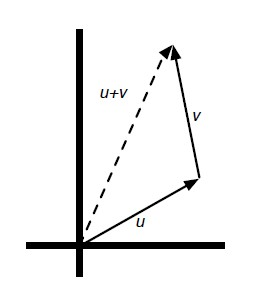# Linear Algebra - Scalar (Multiplication|Product) - Scaling

Scalar Multiplication (Scaling) is the multiplication of a vector (for instance $v_a$ ) by a scalar (real number) (for instance $\alpha$ ) to produce another vector (for instance $v_b$ )

$f (v_a) = \alpha.v_a = v_b$

Multiplying a vector v by a scalar $\alpha$ is defined as multiplying each entry of v by $\alpha$ :

$\alpha.v = \alpha[v_{},v_{},\dots,v_{[n]}] = [\alpha.v_{},\alpha.v_{}, \dots ,\alpha.v_{[n]}]$

$v. \alpha$ is not legal whereas $\alpha . v$ is.

Scalar Multiplication is used to define a line

## Property

Scalar multiplication is:

• Associative: $\alpha(\beta.v) = (\alpha.\beta)v$

## Geometric Representation

The green arrow represents the vector [4, 1.5] and the red arrow represents two times this vector.

• Scalars bigger than 1 give rise to somewhat larger copies of the original vector
• Scalars smaller than 1 give rise to somewhat smaller copies of the original vector
• Negative scalars give rise to vectors pointing in the opposite direction

## Computation

2 [5, 4, 10] = [2 x 5, 2 x 4, 2 x 10] = [10, 8, 20]


Python:

def scalarVectorMult(alpha, v): return [alpha*x for x in v]


Discover MoreGeometry - Scaling

Scaling is a transformation that is generally applied by the transformation matrix See also: The functional form becomes the following matrix. Using the standard transformation matrix notation,...Linear Algebra - (Dot|Scalar|Inner) Product of two vectors

A dot Product is the multiplication of two two equal-length sequences of numbers (usually coordinate vectors) that produce a scalar (single number) Dot-product is also known as: scalar product or...Linear Algebra - Scalar

Real number in linear algebra are called Scalar_(mathematics)scalars and relates to vectors in a vector space through the operation of scalar multiplication. Greek letters () denote scalars.Linear Algebra - Vector Vector Operations

Vector Vector Operations: (also known as addition or substraction) (Scaling) Using operator overloading, you can compute this operations with the following syntax. Operation Syntax...Python - NumPy

NumPy is a Python library for working with arrays. NumPy provides abstractions that make it easy to treat these underlying arrays as vectors and matrices....